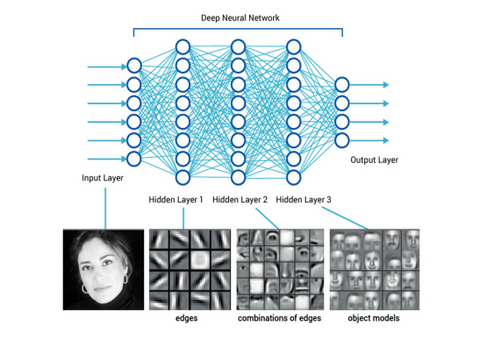# What are Convolutional Neural Networks (CNN)?

A Convolutional Neural Network, or simply CNN, is a particular type of artificial neural network, used primarily for image analysis and the application of graphic filters.

The basic structure and main processes of a CNN are convolution, pooling, the total connection layer, and the learning layer, which are explained as follows:

• The convolution process, characteristic of this type of neural network, is inspired by biological processes for visual analysis in living organisms. The layer of neurons that deals with the convolution divides the image into various overlapping fragments, which are then analyzed to identify the features, transferring the information to the following layer in the form of a feature map containing the relations between neurons and features.
• The pooling process consists of reducing the size of data by generalizing, so as to speed up the analysis without losing too much precision. In the case of an image, the process is very similar to a reduction in pixel quality: a pixel region becomes a single pixel to which a color is assigned based on the average color of its region.
• The total connection layer is the last hidden layer of the neural network, in which all the inputs of the various neurons are put together, allowing you to identify the features.
• The final layer for all neural networks is the learning layer (loss layer) that allows the system to modify the values associated with neurons based on the correctness of the results produced.

The training method most often used is the backpropagation.

Now, let us look at some of the main characteristics of this type of networks can be identified by three key points:

• Three-dimensional volumes of neurons: In CNN levels, the neurons are arranged in three-dimensional structures with a certain width, height, and depth. Each of these structures is connected only to a subset of structures of the previous level, which takes the name of the receptive field.
• Local connections: Thanks to the use of local connections, CNNs exploit the local spatial correlation present in the input data. At each level of the network, specific convolution filters (i.e., the weights of the neurons), which maximize the response to a given local input pattern, are learned. By stacking many levels of this type, thanks to the local connection paradigm, the neurons of the last levels of the network will have a wider receptive field than those of the initial levels. The result is the preliminary creation of a set of representations of small parts of the input (called feature maps) that are then assembled to create an overall representation of larger areas.

An example is shown in the following figure:Deep neural network applied to the face recognition.

• Shared weights: The filters learned at a certain level are replicated across the entire visual field. These replicated units have the same parameters (weights and bias) and form a feature map. This means that all the neurons in a certain level of the network learn to recognize the same feature in the data and thanks to the replication, these features are identified independently from their position in the visual field.

Hope this was helpful. Do check out our weekly blogs here.